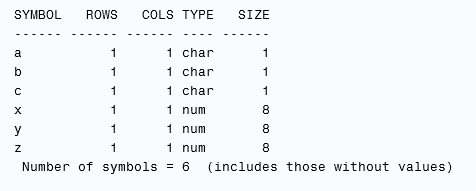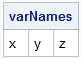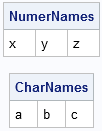The SAS/IML READ statement has a few convenient features for reading data from SAS data sets. One is that you can read all variables into vectors of the same names by using the _ALL_ keyword. The following DATA steps create a data set called Mixed that contains three numeric and three character variables. PROC IML then reads those variables into vectors:

```data Mixed; x=1; y=2; z=3; a='A'; b='B'; c='C'; run;   proc iml; use Mixed; read all var _ALL_; close Mixed; show names;```Notice that the SAS/IML vectors that are created are named x, y, z, a, b, and c—the same names as the variables in the Mixed data set. As mentioned in a previous article, the _ALL_ keyword (in combination with the KEEP and DROP options) provides a powerful way to read data.

However, you need to be aware of a special behavior when you use the _ALL_ keyword in conjunction with the INTO clause. The INTO clause reads variables into a matrix. Recall that a matrix is either character or numeric. You cannot have a matrix that has some numerical elements and some character elements. Consequently, what happens in the following example?

```/* what happens if you use the INTO clause? */ use Mixed; read all var _ALL_ into A[colname=varNames]; close Mixed; print varNames;```As shown in the table, SAS/IML reads the numeric variables into the matrix A. The character variables in the Mixed data set are dropped.

You can read the character variables into a matrix, but you need to use a different keyword. The SAS/IML language supports the _NUM_ and _CHAR_ keywords in order to read all numeric and all character variables (respectively) into a matrix, as shown in the following example:

```use Mixed; read all var _NUM_ into X[colname=NumerNames]; read all var _CHAR_ into C[colname=CharNames]; close; print NumerNames, CharNames;```I always use the _NUM_ or _CHAR_ keyword in conjunction with the INTO statement. It makes the code clearer and easier to read. And there is never any question regarding the type of the matrices that result.

ShareDistinguished Researcher in Computational Statistics

Rick Wicklin, PhD, is a distinguished researcher in computational statistics at SAS and is a principal developer of SAS/IML software. His areas of expertise include computational statistics, simulation, statistical graphics, and modern methods in statistical data analysis. Rick is author of the books Statistical Programming with SAS/IML Software and Simulating Data with SAS.

1.Dear Mr. Rick Wicklin
I realy need to convert a sas data set includes column i , j , Y to a square matrix, how can i do that, i realy so pleased if you help me about that: for example:
i j Y
1 1 45
1 2 35
2 1 28
2 2 32

change to this matrix:
45 35
28 32
and for example when we don't have Y=28 it put Y=35 instade.

•In your example, you have all of the data and the J column changes faster than the I column. In that case, you just need to reshape the vector Y into a matrix:
Y = shape(Y, max(i), max(j));
Fort more complicated examples with missing indices, post your question and example data to the SAS/IML Support Forum.

2.i j Y
1 1 45
1 2 35
2 1 28
2 2 32
3 1 52
3 2 67
change to this matrix:
45 28 52
35 32 67

•Same answer, but then apply the transpose operator Y=T(Y);

3.Hi, I am trying to save the variable names as a vector in iml using following statement:

read all var _num_ into data[colname=Varnames];

In fact it works, but the problem I encounter is that the vector Varnames cuts the length of the variable name. For instance if the name of one variable is "mm_10058", then it saves only "mm_1005".

Any suggestions how I can solve this problem?

•I have never seen the problem that you claim to see. On every example that I run, the variable names are not truncated.

You can ask IML questions at the SAS Communities that are linked to on the sidebar. You can also contact SAS Technical Support and send them the data and the program.

4.Possibly unrelated Question :
I have 2 column variables : Id (character) and Disease (categorical, 12 different categories)
Each Id may have more than 1 Disease observation, which are ordered by Id.

I wish to create a 12x12 matrix for the categorical variable (Disease), in order to cross-tabulate the different diseases.
Ie. display the co-occurance of diseases by participant as a sort of binplot/heatmap/cross-tabulation...
The desired matrix would have x = Disease AND y = Disease

Many thanks

•If you want the solution in Base SAS, post your question to the Base SAS Programming support community. If you want a SAS/IML solution, post to the SAS/IML Support Community. Provide sample data and the output that you expect/

5.sami mushtaq on

Problem:
Write the following functions operating on square matrices (2-dimensional arrays)
of real numbers:
• get – reading the content of a matrix given as its parameter,
• print – printing the content of a matrix given as a parameter,
• sum – calculating a matrix being the sum of the two matrices given as its
parameters,
• product – calculating a matrix being the product of the two matrices given as its
parameters.